# RBSE Maths Class 8 Chapter 11: Important Questions and Solutions

RBSE Maths Chapter 11 – Linear Equations with one variable Class 8 Important questions and solutions can be used as a guide to facing the board exams. All the important questions from previous years, additional questions and solutions covering all the concepts of Chapter 11, available at BYJU’S, contain detailed step by step answers along with explanations. All these important questions are based on the new pattern prescribed by the RBSE. Students can also get the syllabus and textbooks on RBSE Class 8 solutions.

Chapter 11 of the RBSE Class 8 Maths will help the students to solve problems related to solving for an unknown variable, finding solutions to word problems.

### RBSE Maths Chapter 11: Additional Questions and Solutions

Question 1: The necessary condition for a linear equation is that

(a) the highest power of the variable is 1.

(b) the highest power of the variable is 2.

(c) the highest power of the variable is 3.

(d) None of the above.

Question 2: On transposing the term with the sign changed

(a) in ‘x’

(b) in ‘+’

(c) in ‘-’

(d) remains the same

Question 3. The value of x in 3x = 21 is

(a) 7

(b) 24

(c) 18

(d) 83

Question 4: The solution of a linear equation maybe

(a) any natural number

(b) any rational number

(c) any real number

(d) any whole number

Question 5: The solution of x – 4 = 5 is

(a) 4 x 5

(b) 5 x 4

(c) 5 – 4

(d) 5 + 4

### RBSE Maths Chapter 11: Exercise 11.1 Textbook Important Questions and Solutions

Question 1: Solve the following problems.

[i] 6x + 3 = 4x + 11

[ii] 3 (x + 5) = 4x + 9

[iii] 3x + 2 (x + 3) = 21

[iv] [x + 1] /  + [x + 2] /  = [2x – 5] /  + 9

[v] [3x – 2] /  = 4 – {[x + 2] / }

[vi] [x + 2] /  + [x + 4] /  = [x + 6] /  + [x + 8] / 

[vii] 0.6x + 0.25x = 0.45x + 1.2

[viii] 2.5x – 7 = 0.5x + 13

[ix] [7x + 4] / [x + 2] = [- 4 / 3]

[x] [4x + 8] / [5x + 8] = 5 / 6

Solution:

[i] 6x + 3 = 4x + 11

Subtract 3 from both sides

6x + 3 – 3 = 4x + 11 – 3

6x = 4x + 8

Subtract 4x from both sides

6x – 4x = 4x + 8 – 4x

2x = 8

Divide both sides by 2

{2x} / {2} = {8} / {2}

x = 4

[ii] 3 (x + 5) = 4x + 9

Subtract 15 from both sides

3x + 15 – 15 = 4x + 9 – 15

3x = 4x – 6

Subtract 4x from both sides

3x – 4x = 4x – 6 – 4x

-x = -6

x = 6

[iii] 3x + 2 (x + 3) = 21

3x + 2x + 6 = 21

Add similar elements 3x + 2x = 5x

5x + 6 = 21

Subtract 6 from both sides

5x + 6 – 6 = 21 – 6

5x = 15

x = 15 / 5

x = 3

[iv]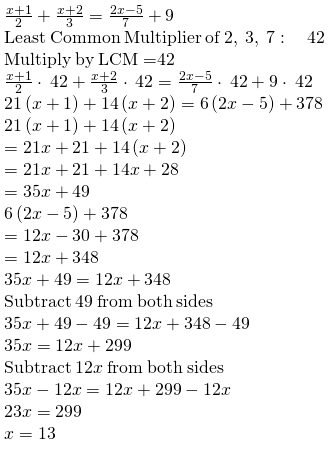[v]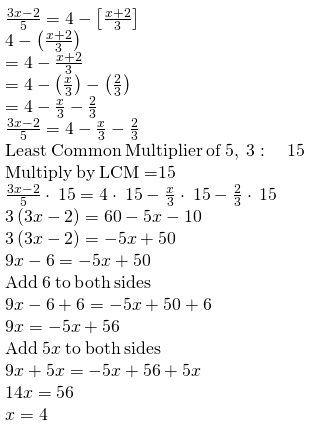[vi]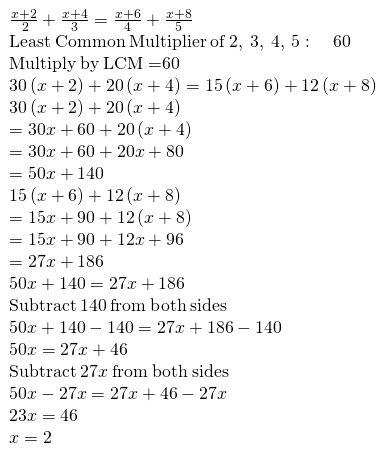[vii]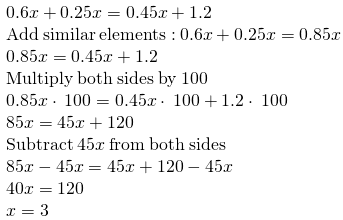[viii]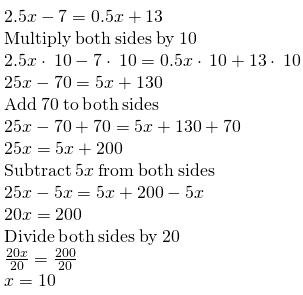[ix]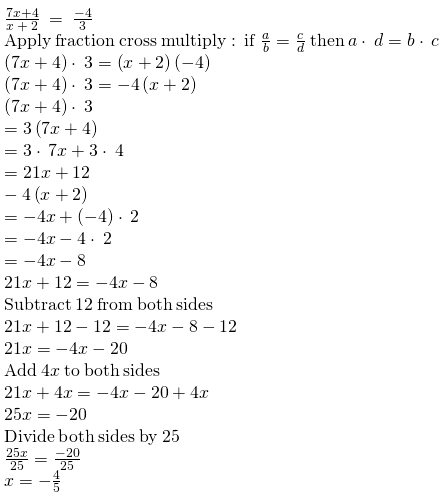[x]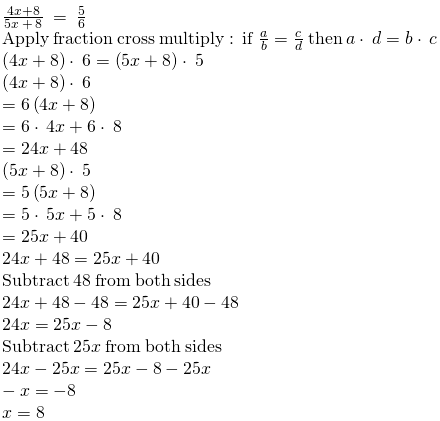### RBSE Maths Chapter 11: Exercise 11.2 Textbook Important Questions and Solutions

Question 1: The numerator of a rational number is less than its denominator by 3. If 5 is added to both i.e. its numerator and its denominator then it becomes ¾. Find the numbers.

Solution:

Let the denominator of rational number be x.

Then, according to the question, the numerator is = x – 3.

Hence, the rational number = [x – 3] / x.

Now, 5 is added to numerator and denominator,

[x – 3 + 5] / [x + 5] = ¾

[x + 2] / [x + 5] = ¾

4 * [x + 2] = 3 * [x + 5]

4x + 8 = 3x + 15

4x – 3x = 15 – 8

x = 7

Denominator = 7

Numerator = 7 – 3 = 4

Required rational number = 4 / 7.

Question 2: What should be added in the numerator and denominator of a fraction [5 / 13] so that the fraction becomes 3 / 5.

Solution:

Let the number be ‘x’.

According to the question,

[x + 5] / [x + 13] = 3 / 5

5 * [x + 5] = 3 * [x + 13]

5x + 25 = 3x + 39

5x – 3x = 39 – 25

2x = 14

x = 14 / 2

x = 7

The number that has to be added in the numerator and denominator of a fraction [5 / 13] so that the fraction becomes 3 / 5 is 7.

Question 3: What should be subtracted from the numerator and denominator of the fraction [15 / 19] so that the fraction becomes 5 / 7.

Solution:

Let the number be ‘x’.

[15 – x] / [19 – x] = 5 / 7

7 * [15 – x] = 5 * [19 – x]

105 – 7x = 95 – 5x

105 – 95 = 7x – 5x

10 = 2x

x = 10 / 2

x = 5

The number that has to be subtracted from the numerator and denominator of the fraction [15 / 19] so that the fraction becomes 5 / 7 is 5.

Question 4: Ramesh distributed his capital, half of the capital to his wife, one third to his son and remaining 50,000/- to his daughter. Find the total amount of his capital.

Solution:

Let ‘x’ be the total capital.

According to the question,

Money given to wife = x / 2

Money given to son = x / 3

Money given to daughter = Rs 50,000

From question,

[x / 2] + [x / 3] + 50,000 = x

Taking least common multiplier we get,

3x + 2x + [50,000 * 6] = 6 * x

5x + 3,00,000 = 6x

6x – 5x = 3,00,000

x = 3,00,000

The required capital is Rs 3,00,000.

Question 5: 5 times of any number is 48 more than its double. Find the numbers.

Solution:

Let the required number be x.

According to the question,

Five times of number = 5x

Twice [double] the number = 2x

5x = 2x + 48

5x – 2x = 48

3x = 48

x = 48 / 3

x = 16

The required number is 16.

Question 6: Distribute 45 in this way that one part is 7 less than three times of another part.

Solution:

Let one part be x.

According to the question second part = 45 – x.

The question says that one part is 7 less than three times another part. If one part is 45 – x, then the second part is given by

x = 3 * (45 – x) – 7

x = 135 – 3x – 7

3x + x = 135 – 7

4x = 128

x = 128 / 4

x = 32

One part = 32

Another part = 45 – x = 45 – 32 = 13.

The required parts are 32 and 13.

Question 7: Age of Ranu is three times of Sujal’s age. After 4 years, the sum of their age will be 40 years. Find their present age.

Solution:

Let x years be the age of Sujal.

Then according to the question, 3x will be the age of Ranu.

After 4 years,

Age of Sujal = (x + 4) years

Age of Ranu = (3x + 4) years

(x + 4) + (3x + 4) = 40

x + 4 + 3x + 4 = 40

4x + 8 = 40

4x = 40 – 8

4x = 32

x = 32 / 4

x = 8

The present age of Sujal is 8 years and Ranu is 3 * x = 3 * 8 = 24 years.

Question 8: The length of a rectangle exceeds its breadth by 6 meters, If its perimeter is 64 meters then find its length and breadth.

Solution:

Let x meter be the breadth of the rectangle.

Then, the length of rectangle = (x + 6) meters.

Perimeter of a rectangle = 2 (length + breadth)

= 2 (x + (x + 6))

= 2 * (2x + 6) meters

According to the question,

2 * (2x + 6) = 64

4x + 12 = 64

4x = 64 – 12

4x = 52

x = 13

The breadth of rectangle = 13 meters.

The length of rectangle = (13 + 6) meters = 19 meters.

Question 9: The sum of the digits of a two-digit number is 12. The new number formed by reversing the digit is greater than the original number by 54. Find the value of the original number.

Solution:

Let the one’s digit be x.

Since the sum of digits is 12, the tens digit = (12 – x) . . . . (i)

Number = 10 * tens digit + ones digit

= 10 * (12 – x)+ x

= 120 – 10x + x

= 120 – 9x

Now, if the digits are reversed, then one’s digit = 12 – x and ten’s digit = x.

New Number = 10 * tens digit + ones digit

= 10 * (x) + 12 – x

= 10x + 12 – x

= 10x – x + 12

= 9x + 12

According to the question,

9x + 12 = 120 – 9x + 54

9x + 12 = 174 – 9x

9x + 9x = 174 – 12

18x = 162

x = 162 / 18

x = 9

One’s digit = 9 and ten’s digit = 12 – 9 = 3

The required number = 39.

Question 10: In a two-digit number, the first digit is four times of the second digit. Adding this to the new number formed by reversing the digits, 110 is obtained. Find the numbers.

Solution:

Let the ones digit be x.

The ten’s digit is 4x.

Number = 10 * tens digit + ones digit

= 10 * (4x) + x

= 40x + x

= 41x

Now the digits are reversed, one’s digit = 4x, and ten’s digit = x

Number = 10 x tens digit + ones digit

= 10 * x + 4x

= 10x + 4x

= 14x

According to the question,

41x + 14x = 110

55x = 110

x = 110 / 55

x = 2

Hence, the one’s digit = 2 and ten’s digit = 4 * 2 = 8

Number = 82

Again, if one’s digit is 4 times of tens digit, then number = 28

Hence, the required can be either 82 or 28.

### RBSE Maths Chapter 11: Additional Questions and Solutions

Question 1: Match the following.

 Question Answer choices 1] The one’s digit is 1 and the tens digit is 2. (a) 0 2] The degree of a linear equation. (b) ⅗ 3] The maximum solutions of a linear equation are (c) 21 4] If the numerator is 3 and the denominator is 5, then the fraction is (d) 1

Solution:

1] (c)

2] (d)

3] (a)

4] (b)

Question 2: Which type of equations can be solved by linear equations?

Solution:

Age problems, number problems, area problems etc. can be solved by linear equations.

Question 3: Solve 2x – 3 = 7.

Solution:

2x – 3 = 7

2x = 7 + 3

2x = 10

x = 5

Question 4: Solve 2x – 3 = x + 2

Solution:

2x – 3 = x + 2

2x – x = 2 + 3

x = 5

Question 5: Solve the equation [x / 3] + 1 = [7 / 15]

Solution:

[x / 3] + 1 = [7 / 15] [x / 3] = [7 / 15] – 1

[x / 3] = [7 – 15] / 15

[x / 3] = [-8 / 15]

x = 3 * [-8 / 15]

x = [-24 / 15]

x = [-8 / 5]

Question 6: Check the equation [15 / 4] – 7x = 9 at x = [-3 /4].

Solution:

[15 / 4] – 7 * [-3 / 4] = 9

[15 / 4] + [21 / 4] = 9

[36 / 4] = 9

9 = 9

LHS = RHS

Hence, the equation [15 / 4] – 7x = 9 at x = [-3 /4] is valid.

Question 7: Solve the equation 4x – [2 + (x – (3 – x))] = 3x + 6

Solution:

4x – [2 + x – 3 + x] = 3x + 6

4x – 2 – x + 3 – x = 3x + 6

2x + 1 = 3x + 6

2x = 3x + 6 – 1

2x – 3x = 6 – 1

-x = 5

x = -5

Question 8: √3x – 2 = 2√3 + 4

Solution:

√3x – 2 = 2√3 + 4

√3x = 2√3 + 4 + 2

√3x = 2√3 + 6

x = [2√3 + 6] / [√3]

x = 2√3 [1 + √3] / √3

x = 2 [1+ √3]

Question 9: Solve the equation [8x + 3] / [2x – 4] = [4x / x – 5]

Solution: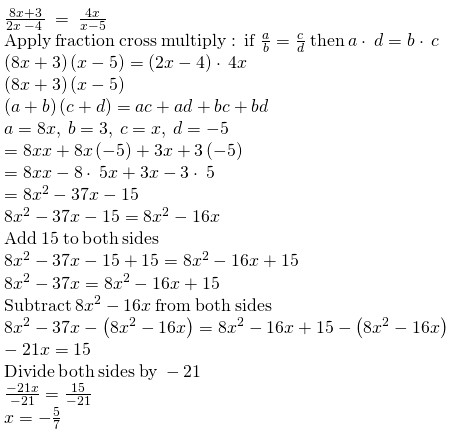Question 10: A bag contains 15 coins of two-rupee and five-rupee. The total amount of the coins is Rs 45. How many coins of each are there in the bag?

Solution:

Let the two-rupee coins in the bag be ‘x’.

Then the 5-rupee coins = 15 – x

Rs 2x is the value of x two rupee coins.

Rs 5(15 – x) is the value of 5-rupee coins.

According to the question,

2x + 5 * (15 – x) = 45

2x + 75 – 5x = 45

2x + 75 – 45 – 5x = 0

-3x + 30 = 0

30 = 3x

x = 30 / 3

x = 10

Hence, the number of 2-rupee coins = x = 10 and number of 5-rupee coins = 15 – x = 15 – 10 = 5.

Question 11: Find the three consecutive numbers where the sum of twice of the first number, thrice of the second number and four times of the third number is 182.

Solution:

Let three consecutive numbers be x, x + 1 and x + 2.

Then according to the question 2x + 3 (x + 1) + 4 (x + 2) = 182

2x + 3x + 3 + 4x + 8 = 182

9x +11 = 182

9x = 182 – 11

9x = 171

x = 171 / 9

x = 19

Hence, the first number = 19, the second number = 19 + 1 = 20 and third number = 19 + 2 = 21.

2 * 19 + 3 (19 + 1) + 4 (19 + 2) = 182.

Question 12: Ramesh’s father is 27 years older than Ramesh. After 5 years, the ratio of ages of Ramesh and his father would be 2:3. Find their present age.

Solution:

Let the age of Ramesh be x years.

Then father’s age = (x + 27) years

After 5 years, the age of Ramesh will be = (x + 5) years

After 5 years, the age of father = x + 27 + 5 = (x + 32) years

According to the question

[x + 5] / [x + 32] = [2 / 3]

3 * [x + 5] = 2 * [x + 32]

3x + 15 = 2x + 64

3x – 2x = 64 – 15

x = 49

Ramesh’s age x = 49 years and his father’s age is x + 27 = 49 + 27 = 76 years.

Question 13: Solve the equation [6x2 + 13x – 4] / [2x + 5] = [12x2 + 5x – 2] / [4x + 3].

Solution: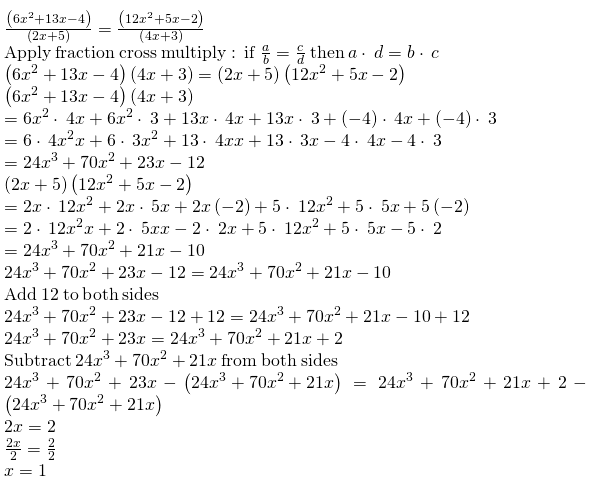Question 14: The denominator of a rational number exceeds its numerator by 5. If 2 is added to its numerator and denominator then we get 1 / 2. Find that rational number.

Solution:

Let the numerator be ‘x’.

According to the question, the denominator of a rational number exceeds its numerator by 5, hence the rational number is [x] / [x + 5].

Now by adding 2 to both numerator and denominator,

[x + 2] / [x + 5 + 2] = [1 / 2] [x + 2] / [x + 7] = [1 / 2]

2 * [x + 2] = 1 * [x + 7]

2x + 4 = x + 7

2x – x = 7 – 4

x = 3

Hence, the numerator = 3, denominator = x + 5 = 3 + 5 = 8

The required rational number = 3 / 8.

Question 15: The length of a rectangle exceeds its breadth by 3 meters. If its perimeter is 54 meters then find its length and breadth.

Solution:

Let the breadth of the rectangle be ‘x’ meters.

Length of rectangle = (3 + x) meters.

Perimeter = 2 (length + breadth)

Perimeter = 2 * ((3 + x) + x} = 2 * (2x + 3) meters

According to the question,

2 * (2x + 3) = 54

2 * 2x + 3 * 2x = 54

4x + 6 = 54

4x = 54 – 6

4x = 48

x = 48 / 4

x = 12

The breadth of rectangle = 12 meters and the length of rectangle = (3 + x ) = (3 + 12) = 15 meters.

Question 16: Find the three consecutive multiples of 11, whose sum is 363.

Solution:

Let the three consecutive multiples of 11 be 11x, 11* (x + 1) and 11 * (x + 2), respectively.

According to the question,

11x + 11* (x + 1) + 11* (x + 2) = 363

11x + 11x + 11 + 11x + 22 = 363

33x + 33 = 363

33x = 363 – 33

33x = 330

x = 330 / 33

x = 10

The three consecutive multiple of 11 are,

11 * x = 11* 10 = 110

11 * (x + 1) = 11 * (10 + 1) = 11 x 11 = 121

11 * (x + 2) = 11 * (10 + 2) = 11 x 12 = 132

The three consecutive multiple numbers of 11 are 110,121 and 132.

Question 17: Practice solving the equation 3x + 9 = 15 and the solution is x = 2.

 On both sides New equation Solution 1] Adding 2 3x + 11 = 17 x = 2 2] Subtracting 3 3x + 6 = 12 x = ____ 3] Multiplying by 2 6x + 18 = _____ x = ____ 4] Dividing by 3 _____ = 5 x = ____

Solution:

 On both sides New equation Solution 1] Adding 2 3x + 11 = 17 x = 2 2] Subtracting 3 3x + 6 = 12 x = 2 3] Multiplying by 2 6x + 18 = 30 x = 2 4] Dividing by 3 x + 3 = 5 x = 2

Question 18: Solve the following.

[i] [2x] / [x + 6] = 1

[ii] 10 = x + 3

[iii] 16 = 7x – 9

[iv] [x + 5] / [x] = 2 / 3

[v] 2x + 5 = 3x + 7

Solution:

[i] [2x] / [x + 6] = 1

2x = 1 * [x + 6]

2x = x + 6

2x – x = 6

x = 6

[ii] 10 = x + 3

10 – 3 = x

7 = x

[iii] 16 = 7x – 9

16 + 9 = 7x

25 / 7 = x

[iv] [x + 5] / [x] = 2 / 3

3 * [x + 5] = x * 2

3x + 15 = 2x

3x – 2x = -15

x = -15

[v] 2x + 5 = 3x + 7

2x – 3x = 7 – 5

-x = 2

x = -2